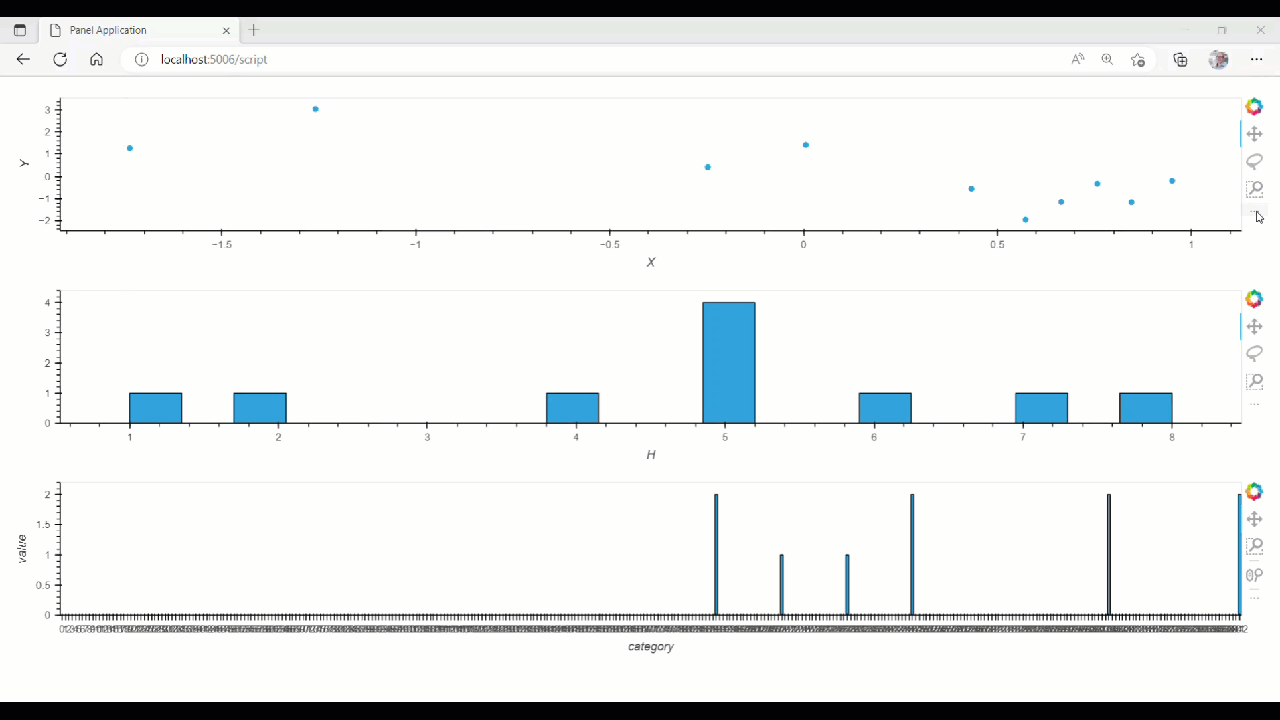# How to extract a selection from a linked selection

I’m still trying to understand how linked selections work, so I might be doing something dumb here, but I’m trying to construct three plots from a large dataframe. The first two can be made easily from an interactive dataframe, but the third requires some manipulation of one of the columns in the dataframe, so I have to apply a function to the dataset to get the right plot. I can link these three plots together with `hv.link_selections`, which is really helpful, but it causes some visual weirdness with the third plot because it doesn’t make sense to plot the unselected data together with the data from the selection. Basically, I’d like the first two plots to maintain the normal linked selection, but for the third plot I’d just like it to be updated each time a new selection is made without trying to visualize data from the old selection. Here’s a code snippet showing what I mean:

``````import holoviews as hv
import pandas as pd
import numpy as np
import panel as pn
import hvplot.pandas

data = {'i' : np.arange(0,10,1),
'X' : np.random.randn(10),
'Y' : np.random.randn(10),
'H' : np.random.randint(0,10,10),
'bars' : np.random.randint(10,20,10)}

df = pd.DataFrame(data)

dfi = df.interactive()
points = dfi.hvplot.points(x='X',y='Y')
hist = dfi.hvplot.hist(y='H')

def getBars(dataset):
mutatedData = dataset.data['bars']*np.random.randint(10,20,10)
bincount = np.bincount(mutatedData)
newDF = pd.DataFrame({'category':range(0,len(bincount)),'value':bincount})
return hv.Bars(newDF, kdims=['category'], vdims=['value'])

dataset = hv.Dataset(df)
bars = dataset.apply(getBars)

pn.Column(ls(points.holoviews()), ls(hist.holoviews()), ls(bars))
``````

This produces the following output:

In my actual usage, the plot doesn’t look quite as bad as what’s shown on the bottom there, but in any case, I’d like the bars plot to just show only data from the newest selection. If there’s some way to extract the selection indices from the linked selections that would be great I’m sure, I just haven’t been able to figure out how to do that. Any help will be greatly appreciated!

Also, I’m really not sure what I’m doing here I guess, but I’m not sure why I can’t stream selections from the terminated interactive object:

``````import holoviews as hv
import pandas as pd
import numpy as np
import panel as pn
import hvplot.pandas
from holoviews.streams import Selection1D

data = {'i' : np.arange(0,10,1),
'X' : np.random.randn(10),
'Y' : np.random.randn(10),
'H' : np.random.randint(0,10,10),
'bars' : np.random.randint(10,20,10)}

df = pd.DataFrame(data)

dfi = df.interactive()
points = dfi.hvplot.points(x='X',y='Y')
hist = dfi.hvplot.hist(y='H')

index_stream = Selection1D(source=points.holoviews())

def getBars(index):
if not index:
data = dataset.data
else: data = dataset.data.iloc[index]
data
mutatedData = data['bars']*np.random.randint(10,20,10)
bincount = np.bincount(mutatedData)
newDF = pd.DataFrame({'category':range(0,len(bincount)),'value':bincount})
return hv.Bars(newDF, kdims=['category'], vdims=['value'])

dataset = hv.Dataset(df)
bars = hv.DynamicMap(getBars, streams=[index_stream])

pn.Column(ls(points.holoviews()), ls(hist.holoviews()), bars)
``````

Any guidance here would be greatly appreciated, I’m clearly missing something.

Trying out your code, I think the main issue is that the object you use as `source` in `index_stream = Selection1D(source=points.holoviews())` is not the same as you show in the panels. `.holoviews()` create a new instance each time.

Futher more the multiplication `mutatedData = data['bars']*np.random.randint(10,20,10)` raises error when you select more than 1 point.

The below code can be used as a working starting point.

``````import holoviews as hv
import pandas as pd
import numpy as np
import panel as pn
import hvplot.pandas
from holoviews.streams import Selection1D

pn.extension(sizing_mode="stretch_width")

data = {'i' : np.arange(0,10,1),
'X' : np.random.randn(10),
'Y' : np.random.randn(10),
'H' : np.random.randint(0,10,10),
'bars' : np.random.randint(10,20,10)}

df = pd.DataFrame(data)
dfi = df.interactive()
points = dfi.hvplot.points(x='X',y='Y', height=200, responsive=True, ).holoviews().opts(default_tools=["box_select"])
hist = dfi.hvplot.hist(y='H', height=200, responsive=True).holoviews()

index_stream = Selection1D(source=points)

def getBars(index):
print(index)
if not index:
data = dataset.data
else: data = dataset.data.iloc[index]

mutatedData = data['bars'].max()*np.random.randint(10,20,10)
bincount = np.bincount(mutatedData)
newDF = pd.DataFrame({'category':range(0,len(bincount)),'value':bincount})
return hv.Bars(newDF, kdims=['category'], vdims=['value']).opts(height=200, responsive=True)

dataset = hv.Dataset(df)
bars = hv.DynamicMap(getBars, streams=[index_stream])As far as I can see you are not using `.interactive` for anything. So you might just use the `df` dataframe instead. Then you don’t need the `.holoviews()` any more.